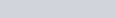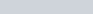• 41
•
• 2
•
• 3
•
•
•
46
Shares

# MAGNETIC FIELD DUE TO STRAIGHT CURRENT-CARRYING CONDUCTOR

### INTRODUCTION

We know that static electric charges create an electric field around itself whose magnitude depends on the value of charge and the distance of the point where we want to find the electric field.

But we also know that when these charges start moving with constant acceleration then it creates an electric field as well as a magnetic field. The produced electric field and magnetic field are perpendicular to each other. The combined motion of the electric field and the magnetic field is called electromagnetic waves.

The magnitude of the magnetic field is proportional to the value of the electric current passing through the wire and the distance of the point where we want to find the magnetic field and its value also depends on the angle between the direction of the current element and the distance vector of the point. See figure below:

The magnetic field is a vector quantity because it has both magnitude and direction. It is denoted by the capital letter B. Its SI unit is Tesla but sometimes Weber and gauss are also used as units of the magnetic field.## MAGNETIC FIELD DUE TO STRAIGHT CURRENT-CARRYING CONDUCTORFig. 2, demonstration of the magnetic field in straight conductor, source: topper learning

We know the current-carrying conductor produces a magnetic field around itself. This magnetic field around the wire seems like a concentric cylinder as a whole but if we take one plane of their cross-section then it appears as a concentric circle, see figure above.

Let’s understand the behavior of the magnetic field around a straight current-carrying conductor through an experiment/activity.

### MATERIALS REQUIRED

You need these materials to perform this activity.

• A cardboard
• Some length of wire
• Ammeter
• Battery
• Iron filings

## PROCEDURE

• Connect the positive terminal of the battery to the positive terminal of the ammeter as shown above.
• Connect the wire to the negative terminal of the ammeter to the negative terminal of the battery.
• Insert the wire from the center of the plane cardboard before connecting it to the battery.
• Keep the middle wire straight and named it X and Y, as shown above.

Now your experimental setup is ready. Supply electric current in the wire. When electric current starts flowing through the wire then sprinkle some iron filings on the cardboard and give some little jerk to the cardboard with your fingers.

Now, what did you see on the cardboard? Did you see any patterns on the cardboard? And how this pattern is looking? You must have seen a pattern like as seen in the figure below.Fig. 3, alignment of iron filings on the cardboard in the influence of magnetic field (), source: nzdl.org

When you sprinkle iron filings on the plane of the cardboard and after giving some gentle jerk, it tries to align in the direction of the magnetic field produced by the electric current and the direction of the magnetic field is perpendicular to the conductor.

The direction of the magnetic field around the straight current-carrying conductor is given by the right-hand thumb rule.

According to this rule – when you catch the wire in your fist such that your thumb points the direction of the electric current then your curled fingers denote the direction of the magnetic field around the wire. See figure below:

Alignment of iron filings shows that produced magnetic field forms a concentric circle around the wire. And this magnetic field lines in the plane of the cardboard and is perpendicular to the conductor.

 If the gaps between the concentric circles are small then it shows a strong magnetic field and if it is large then it shows a weak magnetic field.

## CHARACTERISTICS OF MAGNETIC FIELD DUE TO STRAIGHT CURRENT-CARRYING CONDUCTOR

The characteristics of the magnetic field produced due to a current-carrying conductor are listed below:

• It forms concentric circles around the conductor.
• It lies in a plane and perpendicular to the conductor.
• If the direction of current flow reverses then the direction of the magnetic field is also reversed.
• The strength of the field is directly proportional to the magnitude of the current. This means a high electric current produces a strong magnetic field and a low electric current produces a weak magnetic field.
• The strength of the field at any point is inversely proportional to the distance of the point from the wire.

Watch this video for more understanding.

Stay tuned with Laws Of Nature for more useful and interesting content.